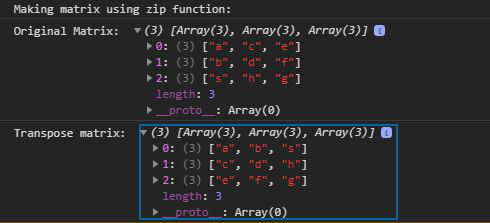Related Articles
D3.js transpose() Function
• Last Updated : 19 Jul, 2020

The transpose() function in D3.js is used to return the transpose of a 2D array. This function helps to visualize the data and making graphs and charts.

Syntax:

```d3.transpose(Matrix);
```

Parameters: This function accept a single parameter as mentioned above and described below:

• Matrix: This parameter holds a matrix as a 2d array

Return value: It returns an array or the matrix.

Below given are a few examples of transpose function.

Example 1: Without using zip function to form a matrix.

## HTML

 ` ` `<``html` `lang``=``"en"``> ` `<``head``> ` `  ``<``meta` `charset``=``"UTF-8"``> ` `  ``<``meta` `name``=``"viewport"`  `        ``content``=``"width=device-width, initial-scale=1.0"``> ` `  ``<``title``>D3.js transpose() Function ` ` ` `<``body``> ` `  ``` `  ``<``script` `type` `= ``"text/javascript"`  `          ``src` `= ``"https://d3js.org/d3.v4.min.js"``> ` `  `` ` `  ``<``script``> ` `    ``console.log(d3.transpose([[1,2,3],[4,5,6]])) ` `  `` ` ` ` ``

Output:Example 2: Using zip function to form a matrix.

## HTML

 ` ` `<``html` `lang``=``"en"``> ` `<``head``> ` `  ``<``meta` `charset``=``"UTF-8"``> ` `  ``<``meta` `name``=``"viewport"`  `        ``content``=``"width=device-width, initial-scale=1.0"``> ` `  ``<``title``>D3 transpose() Function ` ` ` `<``body``> ` `   `  `  ``` `  ``<``script` `type` `= ``"text/javascript"`  `          ``src` `= ``"https://d3js.org/d3.v4.min.js"``> ` `  `` ` `  ``<``script``> ` `    ``console.log("Making matrix using zip function: ") ` `    ``let m=d3.zip(["a","b","s"],["c","d","h"],["e","f","g"]); ` `    ``console.log("Original Matrix: ",m) ` `    ``console.log("Transpose matrix: ",d3.transpose(m)) ` `  `` ` ` ` ``

Output:Supported Browsers: